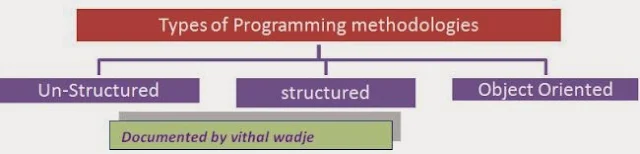Featured

# Programming Methodologies

#### What is Programming Methodology?

A programming methodology is nothing but a type of technique to solve some given requirements using programming languages. For example, we want to add two numbers that are 10 and 20.

Then the example above is an example of a problem because it is very difficult to calculate these types of calculations manually again and again, so to solve the preceding given requirement programmatically with a permanent solution, each programming language has its own methodology and style of coding to solve the given problem. The following are some of the common types of programming methodologies.#### Unstructured Programming

A programming language in which the entire logic of the program is written as a single continuous (nonstop or unbroken) block is called unstructured programming. The following are the key points of unstructured programming languages:
• It is a type of problem-solving technique in which we solve the problem in terms of lots of codes.
• If the requirements increase, then the code is also increased.
• There is no re-usability of existing code.
• Finding an error in a program is very difficult.
• The syntax and structure is very difficult to understand and remember.
• Modification is very difficult.
• Non-structured languages allow only basic data types, such as numbers, strings and arrays.
• Statements are usually one in each line.
Example

JOSS, FOCAL, MUMPS, TELCOMP, COBOL, FORTRAN etc.

#### What is Structured Programming Language?

A programming language in which the entire logic of the program is written by dividing it into smaller units or modules is called a Structured Programming Language.

key points:

• The syntax and structure is very simple to understand and remember.
• Finding an error in a program is easy.
• Modification is easy.
• There is no re-usability of existing code as much as expected.
Example

C

#### What Object Oriented Programming ?

Object Oriented Programming (OOP) is the programming methodology in which each entity is an object.

• Re-usability of existing code.
• Extensibility of existing code.
• Security.
• Flexibility.
• Maintainability Key points of OOP languages
• Classes
• Objects.
• Methods
• Abstraction.
• Encapsulation
• Inheritance
• Polymorphism
Example

C++, Java, C#, F#, VB.NET etc.